# 《算法图解》读书笔记

## 第一章 算法简介

### 二分查找

log_2^n = a  →  2^a=n


// 二分法查找
const binarySearch = (list, target) => {
let min = 0;
let max = list.length - 1;
while (min <= max) {
const middle = Math.floor((min + max) / 2);
if (list[middle] > target) {
max = middle - 1;
} else if (list[middle] < target) {
min = middle + 1
} else {
return middle;
}
}
return null
};

console.log(binarySearch([1, 3, 5, 7, 9], 3));


let a = ['x', 'y'];

// 等同于
{
0: 'x',
1: 'y',
length: 2
}


let a = [1, 2];
a[2.5] = 100;

console.log(a);
// [1, 2, 2.5: 100]


### 运行时间

$O(\log n)$$O(n)$快，当需要搜索的元素越多，前者比后者快得就越多

### 大O表示法

• $O(\log n)$，对数时间，例如二分查找
• $O(n)$，线性时间，例如简单查找
• $O(n * \log n)$，例如快速排序，一种比较快的排序算法
• $O(n^2)$，例如选择排序，一种比较慢的排序算法
• $O(n!)$，例如旅行商问题的解决方案，一种非常慢的算法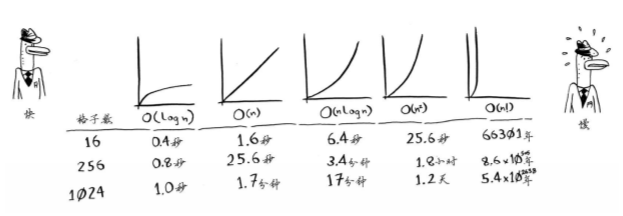## 第二章 选择排序

### 数组

1. 浪费内存
2. 预留的内存用完后，还需要转移元素

### 数组和链表的比较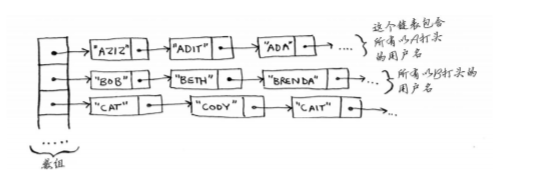### 选择排序

function chooseSort (arr) {
for (let i = 0; i < arr.length; i++) {
let minIndex = i;
for (let j = i; j < arr.length; j++) {
if (arr[j] < arr[minIndex]) {
minIndex = j
}
}
[arr[i], arr[minIndex]] = [arr[minIndex], arr[i]];
}
return arr;
}


## 第三章 递归

### 栈

1. 改用循环
2. 使用尾递归（尾递归的实现需要确保最后一步只调用自身。做到这一点的方法，就是把所有用到的内部变量改写成函数的参数）

## 第四章 快速排序

### 分治法

1. 找出基线条件（即递归终止的条件），这种条件必须尽可能简单
2. 不断将问题分解（或者说缩小规模），直到符合基线条件

// 找到一个矩形可以均匀的划分的最大的小方块的尺寸
const getMaxSquare = (length, width) => {
if (width > length) {
[width, length] = [length, width]
}
if (length % width === 0) {
return width
}
if (length === width) {
return length;
}
return getMaxSquare(width, length - width)
};


tips: 编写设计数组的递归函数时，基线条件通常是数组为空或者只包含一个元素。

### 快速排序

1. 小于基准值的数字组成的数组（无序）
2. 基准值
3. 大于基准值组成的数组（无序）

const quickSort = (arr) => {
if (arr.length < 1) {
return arr
}
const middleIndex = Math.floor(arr.length / 2);
const middle = arr.splice(middleIndex, 1);
const left = [], right = [];
for(let i = 0; i< arr.length; i++) {
(middle > arr[i] ? left : right).push(arr[i])
}
return quickSort(left).concat(middle, quickSort(right))
};


### 再谈大$O$表示法

c是算法所需要的固定时间量，被称为常量。通常不考虑这个常量，因为如果算法的大$O$运行时间不同，这种常量将无关紧要。

## 第五章 散列表

### 散列函数

1. 每次输出的一致性
2. 将不同的输入映射到不同的数字

### 散列表

• 模拟映射关系
• 防止重复
• 缓存、记住数据

JavaScript中的对象结构就是语言实现了的散列表结构。

## 第六章 广度优先搜索（BFS)

### 图算法

1. 使用图来建立问题模型
2. 使用广度优先搜索解决问题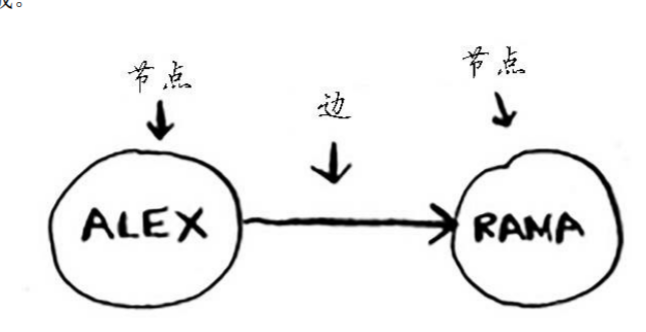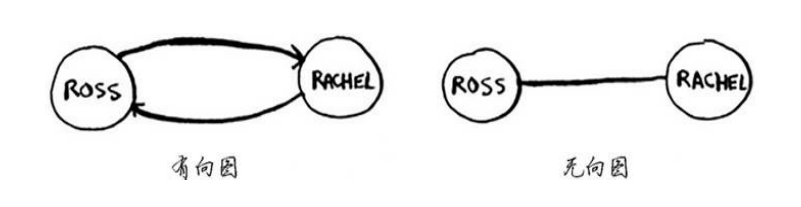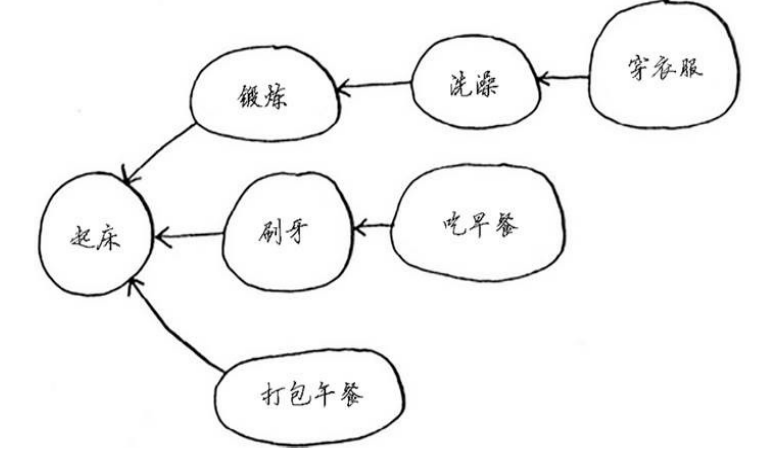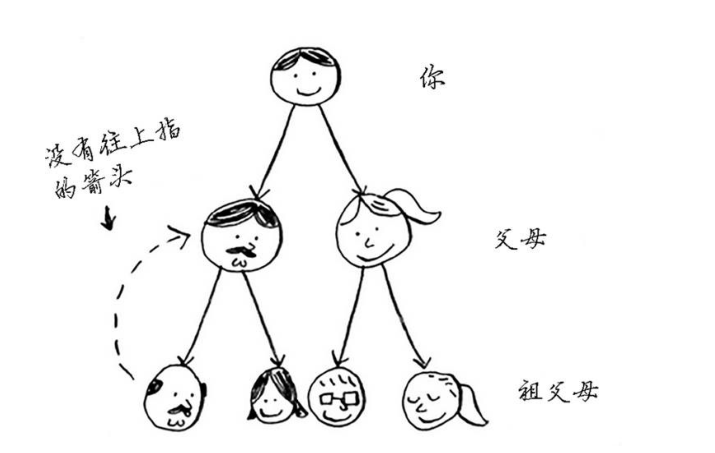### 广度优先搜索

1. 从节点A出发，有前往节点B的路径吗
2. 从节点A出发，前往节点B的哪条路径最短

## 第七章 迪克斯特拉算法

### 使用迪克斯特拉算法

1. 找出“最便宜”的节点，即可在最短时间内到达的节点
2. 更新该节点的邻居的开销
3. 重复这个过程，直到对图中每个节点都这样做了
4. 计算最终路径

### 迪克斯特拉算法的实现

1. 用于存储节点、节点的邻居以及前往节点邻居的开销graph
2. 用于存储每个节点的最短开销costs
3. 用于存储每个节点最短开销情况下的父节点parents
4. 用于存储已经遍历过的节点processed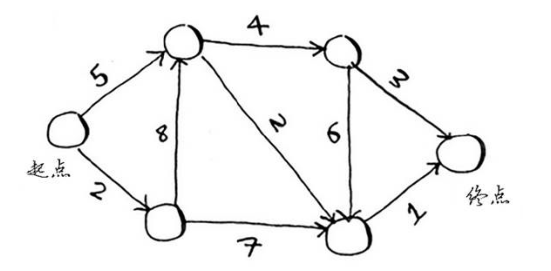// 用于存储节点、节点的邻居以及前往节点邻居的开销
const graph = {
start: {a: 5, b: 2},
a: {c: 4, d: 2},
b: {a: 8, d: 7},
c: {d: 6, end: 3},
d: {end: 1},
};


// 用于存储每个节点的最短开销
const costs =  {
a: 5,
b: 2,
c: Infinity,
d: Infinity,
end: Infinity,
};


// 用于存储每个节点最短开销情况下的父节点
const parents = {
a: 'start',
b: 'start',
c: '',
d: '',
end:'',
};


// 找到开销最小的，并且没有遍历过的节点
const findLowestCostNode = (costs, processed) => {
// 筛选出没有遍历过的节点
const unProcessed = Object.keys(costs).filter(key => !processed[key]);
// 如果存在没有遍历过的节点
if (unProcessed && unProcessed.length > 0) {
// 找出开销最小的节点
return unProcessed.sort((a, b) => costs[a] - costs[b])
}
// 不存在没有遍历过的节点时
return null;
};

// 生成加权图中的最短路径
const getFullPath = parents => {
let key = 'end';
let path = [];
while (key !== 'start') {
path.unshift(key);
key = parents[key];
}
path.unshift('start');
return path.join('→')
};

// 迪科特斯拉算法
const dijkstra = (graph, costs, parents) => {
// 记录所有遍历过的节点
let processed = {};
// 找到开销最小的，并且没有遍历过的节点
let node = findLowestCostNode(costs, processed);
// while 循环在所有节点都遍历过后结束
while (node) {
// 找到当前节点的所有相邻节点
let neighbors = graph[node];
// 找到到达当前节点的开销
let cost = costs[node];
// 对所有相邻节点进行遍历
for (const neighborNode in neighbors) {
// for...in 的安全性检查
if (neighbors.hasOwnProperty(neighborNode)) {
// 找出新的到达相邻节点的开销
const newCost = cost + neighbors[neighborNode];
// 如果计算出的新的开销小于已记录的开销，则进行更新
if (newCost < costs[neighborNode]) {
// 更新开销
costs[neighborNode] = newCost;
// 更新父节点
parents[neighborNode] = node;
}
}
}
// 将当前节点标记为已经处理过
processed[node] = true;
// 找出接下来要处理的节点，并循环，直到所有节点都被处理过
node = findLowestCostNode(costs, processed);
}
// 返回最终的开销和路径
return {
cost: costs['end'],
path: getFullPath(parents),
}
};


{ cost: 8, path: 'start→a→d→end' }


## 第八章 贪婪算法

### 教室调度问题

1. 选出最早结束的课
2. 选择第一堂课结束后的，并且结束最早的课
3. 重复上面的步骤

### 背包问题

// 贪婪算法求解背包问题近似解
const knapsackProblem = () => {
const bagSize = 4;
const items = [
{name: 'sound', size: 4, value: 3000},
{name: 'laptop', size: 3, value: 2000},
{name: 'guitar', size: 1, value: 1500},
{name: 'iphone', size: 1, value: 2000},
];

let value = 0;
let names = [];
let remainSize = bagSize;

// 从价值最大的开始装，能装多少算多少
items.sort((a, b) => b.value - a.value).forEach(v => {
if (v.size <= remainSize) {
value += v.value;
names.push(v.name);
remainSize = remainSize - v.size;
}
});

return {
value, names
};
};


### 集合覆盖问题

1. 列出每个可能的广播台的集合，被称为幂集，可能的子集有$O(2^n)$
2. 在这些集合中，选出覆盖全部50个州的最小集合

### 近似算法

1. 选出这样一个广播台，它覆盖了最多的未覆盖州（即便这个广播台覆盖了一些已覆盖的州，也没有关系）
2. 重复第一步，直到覆盖了所有的州

1. 速度有多快
2. 得到的近似解与最优解的接近程度

### NP完全问题

• 当有1个城市时，可能的路线有1
• 当有2个城市时，可能的路由先2
• 当有3个城市时，可能的路由先6
• 当有4个城市时，可能的路由先24

### 如何识别NP完全问题

• 元素较少时算法的运行速度非常快，但随着元素数量增加，速度会变得非常慢
• 涉及“所有组合”的问题通常是NP完全问题
• 不能将问题分成小问题，必须考虑各种可能的情况，这可能是NP完全问题
• 如果问题涉及序列（如旅行商问题的城市序列）且难以解决，它可能就是NP完全问题
• 如果问题涉及集合（如广播台集合）且难以解决，它可能就是NP完全问题
• 如果问题可转换为集合覆盖问题或旅行商问题，那它肯定是NP完全问题

## 第九章 动态规划

• 每种动态规划解决方案都涉及网格
• 单元格中的值通常就是要优化的值
• 每个单元格都是一个子问题

• 单元格中的值是什么
• 如何将这个问题划分为子问题
• 网格的坐标轴是什么

### 背包问题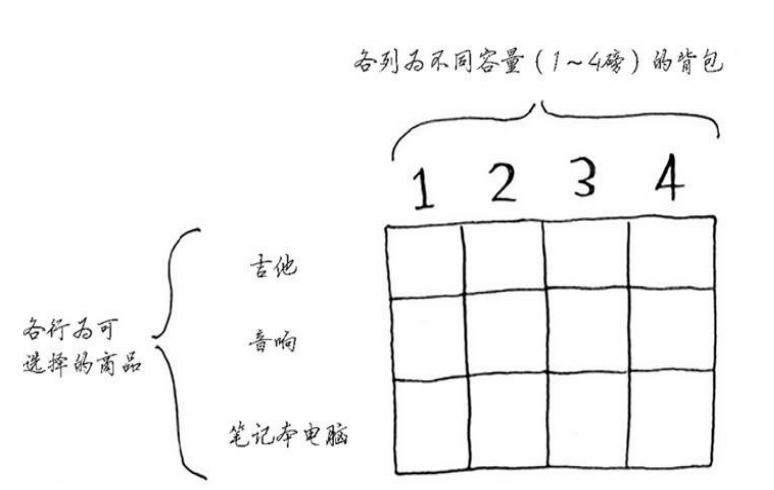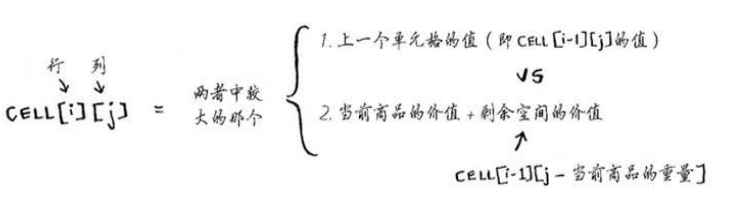（感觉这个代码是为了这个算法生凑出来的，其实关键点把握住就行了，一是上面的公式，而是通过求解子问题，然后通过合并来求解更大问题）

const knapsackProblem = () => {
const bagSize = 4;
const items = [
{name: 'sound', size: 4, value: 3000},
{name: 'laptop', size: 3, value: 2000},
{name: 'guitar', size: 1, value: 1500},
];

// 存储结果的表格
const cell = [];

// 空格子
class CellClass {
constructor() {
this.value = 0;
this.names = [];
}
}

// 外层遍历物品
for (let i = 0; i < items.length; i++) {
// 声明新的一行
cell[i] = [];
// 针对的物品
const item = items[i];
// 当前商品的价值
const currentValue = item.value;

// 内层遍历重量
for (let j = 0; j <= bagSize; j++) {
// 当前格子装入物品后的剩余空间
const remainSize = j - item.size;
// 上一个单元格
const lastCell = (cell[i - 1] && cell[i - 1][j]) ? cell[i - 1][j] : new CellClass();
// 上一个单元格的值（之前的最大值）
const lastCellValue = lastCell.value;

// 如果能装下这个物品
if (remainSize >= 0) {
// 剩余空间对应的单元格
const remainCell = (cell[i - 1] && cell[i - 1][remainSize]) ?cell[i - 1][remainSize]: new CellClass();
// 剩余空间的价值
const remainValue = remainCell.value;
// 预期的最大值
const currentMaxValue = currentValue + remainValue;

// 如果预期的最大值更大
if (currentMaxValue > lastCellValue) {
cell[i][j] = {value: currentMaxValue, names: [item.name, ...remainCell.names]}
} else {
cell[i][j] = {value: lastCellValue, names: lastCell.names}
}
} else {
// 装不下这个物品则取上一个单元格
cell[i][j] = lastCell
}
}
}
console.log(cell);

return cell[items.length - 1][bagSize]
};


### 最长公共子串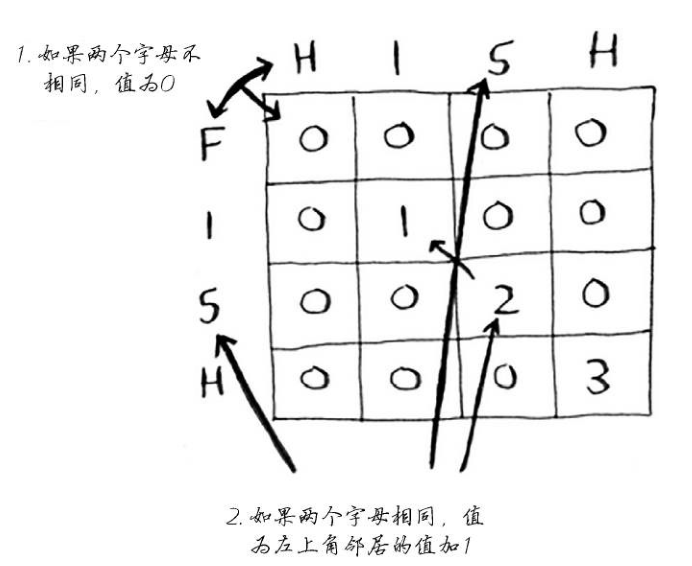const maxSubstring = (string1, string2) => {
let result = {
value: 0,
string: ''
};
let cell = [];
for (let i = 0; i < string1.length; i++) {
cell[i] = [];
for (let j = 0; j < string2.length; j++) {
if (string1[i] === string2[j]) {
const lastCell = cell[i - 1] && cell[i - 1][j - 1];
cell[i][j] = {
value: lastCell ? lastCell.value + 1 : 1,
string: lastCell ? lastCell.string + string1[i] : string1[i]
}
} else {
cell[i][j] = {value: 0, string: ''};
}
if (result.value < cell[i][j].value) {
result.value = cell[i][j].value;
result.string = cell[i][j].string;
}
}
}
return result
};


### 最长公共子序列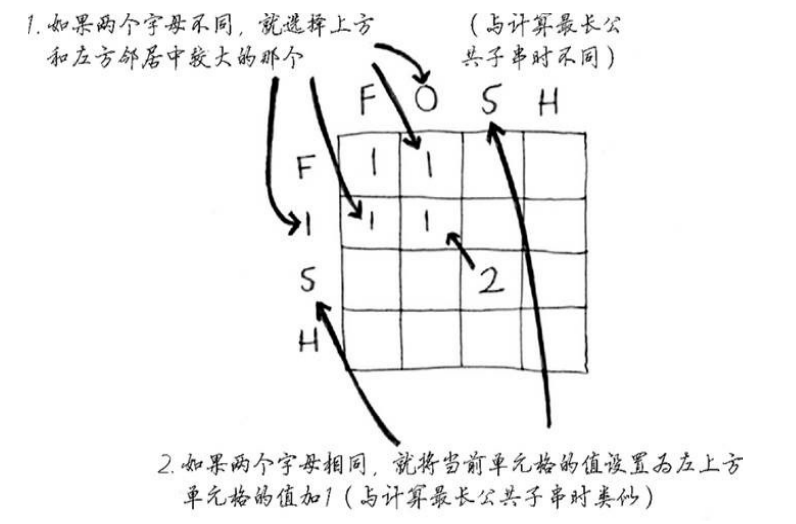// 最长公共子序列
const maxSubsequence = (string1, string2) => {
let result = {
value: 0,
string: '',
};

const cell = [];

for (let i = 0; i < string1.length; i++) {
cell[i] = [];
for (let j = 0; j < string2.length; j++) {
if (string1[i] === string2[j]) {
const lastCell = cell[i - 1] && cell[i - 1][j - 1];
cell[i][j] = {
value: lastCell ? (lastCell.value + 1) : 1,
string: lastCell ? (lastCell.string + string1[i]) : string1[i],
};
// 更新结果
if(cell[i][j].value > result.value) {
result = cell[i][j]
}
} else {
const lastRowCell = cell[i] && cell[i][j - 1] || { value: 0, string: '',};
const lastColCell = cell[i - 1] && cell[i - 1][j]|| { value: 0, string: '',};
if (lastRowCell.value > lastColCell.value) {
cell[i][j] = {
value: lastRowCell.value,
string: lastRowCell.string,
}
} else {
cell[i][j] = {
value: lastColCell.value,
string: lastColCell.string,
}
}
}
}
}

return result;
};


### 小结

• 需要在给定约束条件下优化某种指标时，动态规划很有用
• 问题可以分解为离散的子问题时，可以使用动态规划来解决
• 每种动态规划解决方案都涉及网格
• 单元格中的值通常是你要优化的值
• 每个单元格都是一个子问题，需要考虑如何将问题分解为子问题
• 没有放之四海而皆准的计算动态规划解决方案的公式

## 第十章 K最近邻算法

K最近邻算法（k-nearest neighbours, KNN）简单的说就是在对一个事物分类时，如果自身没有准确的特征值用来分类，就可以看距离它最近的邻居，借此对目标分类。

### 创建推荐系统

（1）特征抽取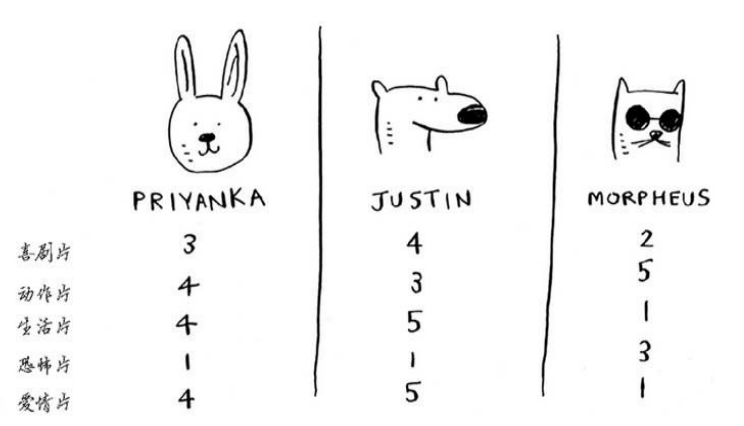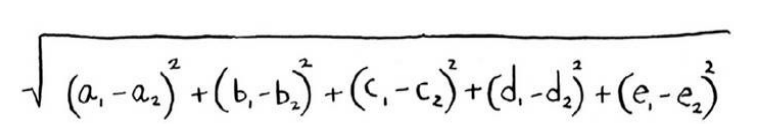（2）回归

• 分类就是编组
• 回归就是预测结果（比如一个数字）

（3）挑选合适的特征值没有通用的准则，必须考虑各种需要考虑的因素

### 机器学习

（1）OCR，光学字符识别，要自动识别字符，可以使用KNN

• 浏览大量的图像，将这些数字的特征提取出来（也就是训练）
• 遇到新图像时提取该图像的特征，再找出它最近的邻居

（2）垃圾邮件过滤器

## 第十一章 接下来如何做

### 树

1. 左子树的所有节点的值都小于或等于它的根节点的值
2. 右子树上所有节点的值均大于或等于它的根节点的值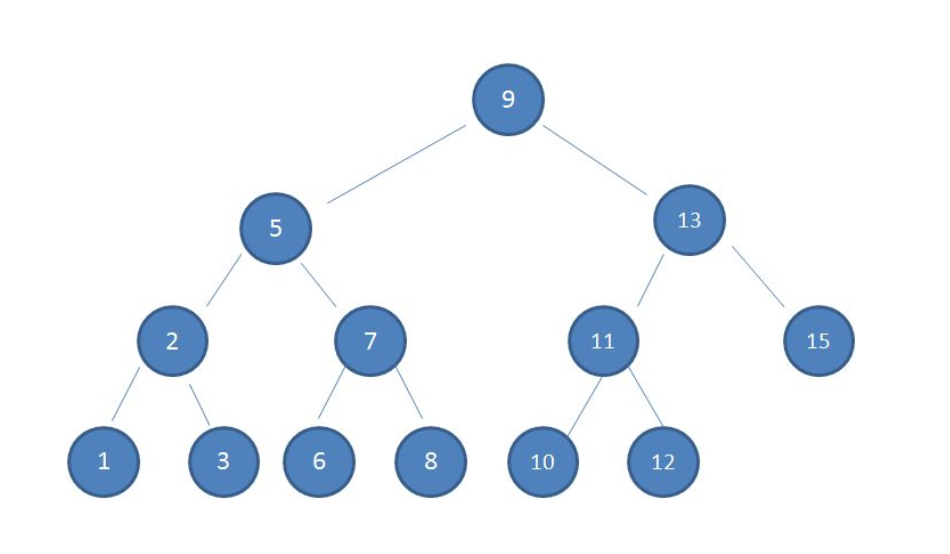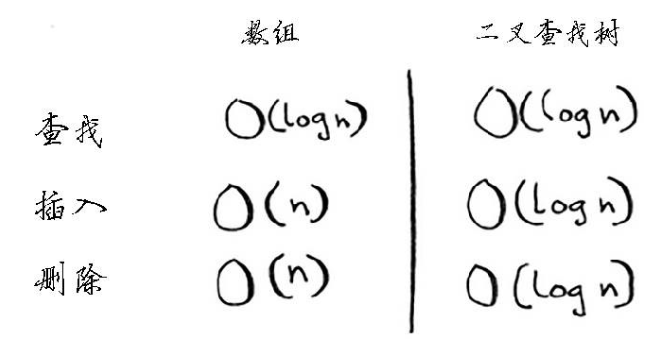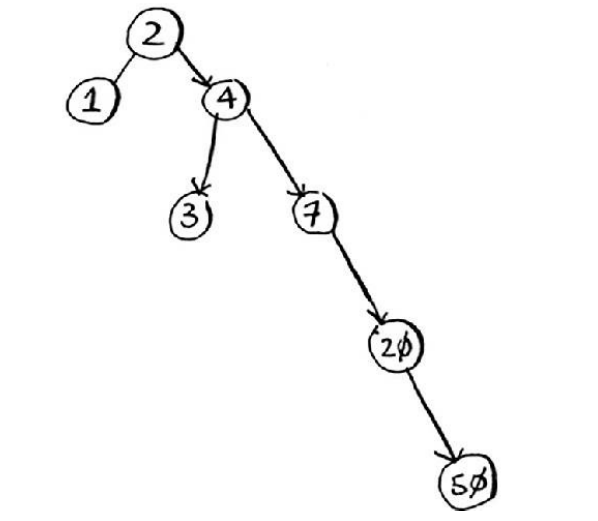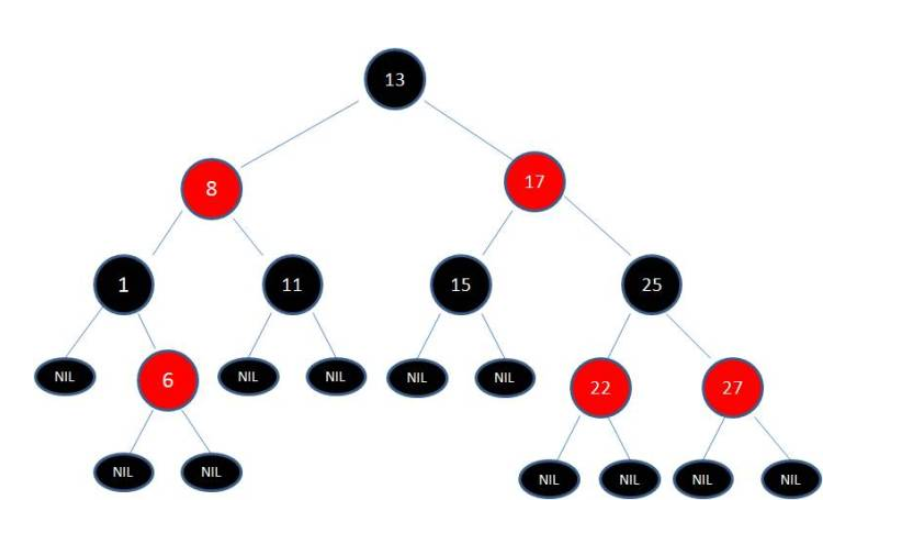### 傅里叶变换

（1）傅立叶级数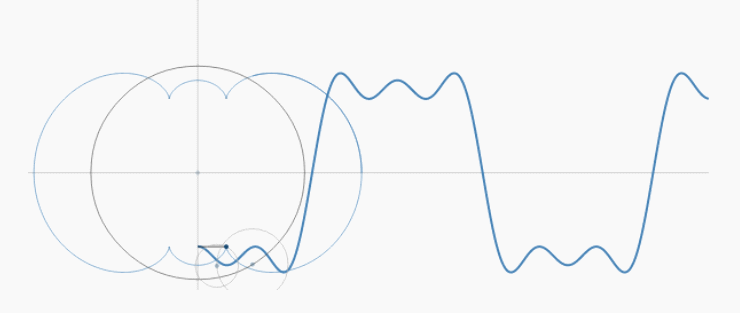（2）傅里叶变换

### MapReduce

MapReduce是第一代计算引擎，Tez和Spark是第二代。MapReduce的设计，采用了很简化的计算模型，只有Map和Reduce两个计算过程（中间用Shuffle串联），用这个模型，已经可以处理大数据领域很大一部分问题了。

### 布隆过滤器和HyperLogLog

HyperLoglog是一种类似于布隆过滤器的算法。可以近似地计算集合中不同的元素数，提供不精确的去重计数，它也不能给出准确的答案，但是八九不离十，而占用的内存空间却少得多。关于HypoerLogLog的解释可以阅读这篇文章

### SHA算法

SHA函数（secure hash algorightm）是一种安全散列算（也就是哈希算法），给定一个字符串，SHA返回其散列值。

（1）比较文件

（2）检查密码

SHA能在不知道原始字符串的情况下进行比较，进行密码登陆时，不会直接存储密码，而是比较散列值。网站保存的不是原始密码，而是密码的散列值。

SHA散列算法是单项的，可以根据字符串计算散列值，但是无法根据散列值推出原始字符串。

SHA实际上是一系列散发：SHA-0/SHA-1/SHA-2/SHA-3，SHA-0和SHA-1被发现存在一些缺陷，应该使用SHA-2和SHA-3来计算密码散列值，最安全的散列函数是bcrypt。

### 局部明暗的散列算法

SHA算法是局部不敏感的，对一个字符串，如果只改动了其中一个字符，得到的两个散列值是完全不同的，这就让攻击者无法通过比较散列值是否类似来破解密码。

• 论文查重/资源查重

### Diffie-Hellman密钥交换

Diffie-Hellman密钥交换解决了两个问题：

1. 双方无序知道加密算法，不必会面协商使用的加密算法
2. 破解加密非常困难

### 线性规划©️2019 CSDN 皮肤主题: 编程工作室 设计师: CSDN官方博客